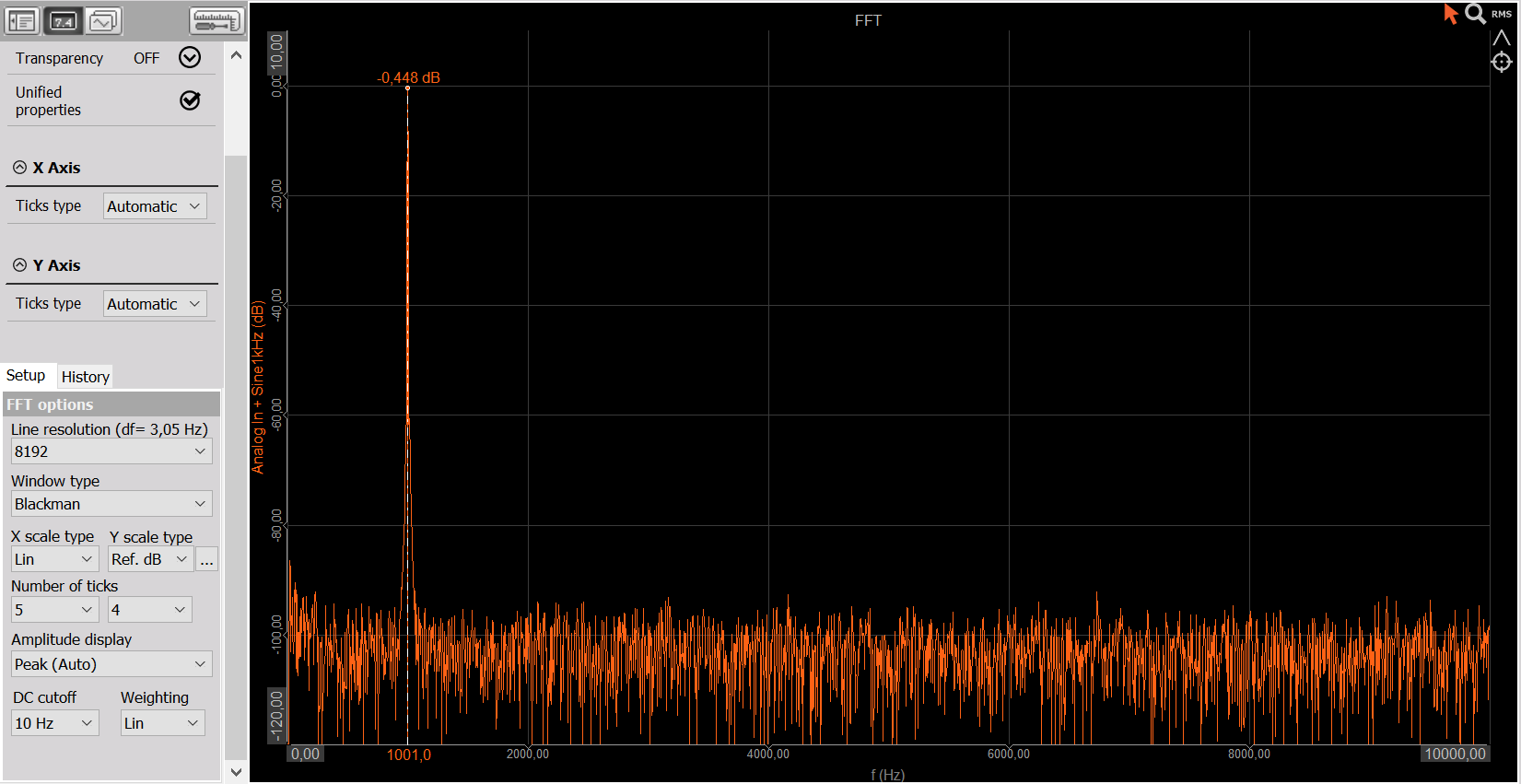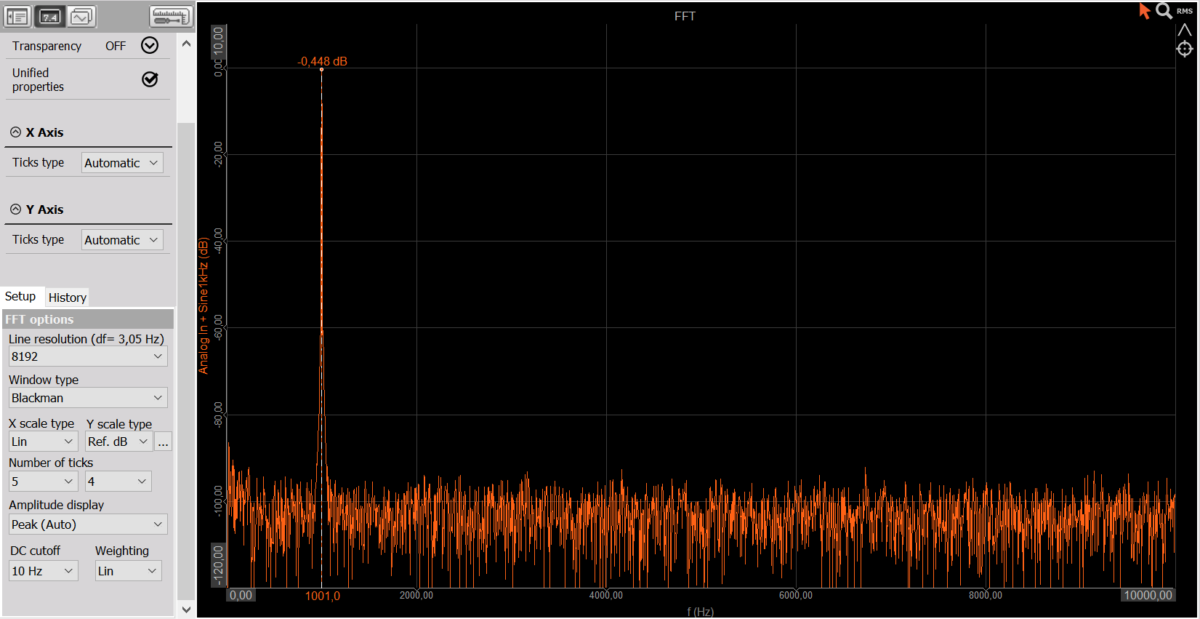Measurement basics – signal to noise ratio

Let’s look at how to measure signal to noise ratio of a signal with MonoDAQ-U-X hardware and DEWESoft software. Signal to noise ratio is a value that tells us the ratio between the meaningful information in a signal and the noise  that is obscuring this information. Technically it is expressed in the ratio of the signal power to the noise power.

We will wire the analog output channel of a MonoDAQ-U-X device to one of its analog input channels. This way we can generate the signal and measure it, which will be handy for this learning exercise. Channel setup table of the MonoDAQ-U plugin in DEWESoft software should look like this: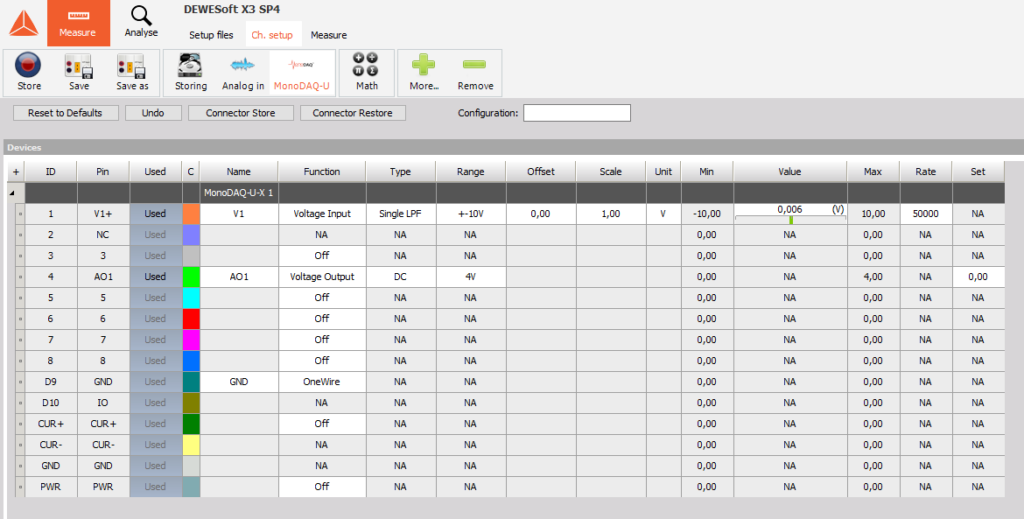The wiring of the output channel to the input channel is simply done by shorting Pin 4 to Pin 1 since both channels are single ended and therefore share the same ground.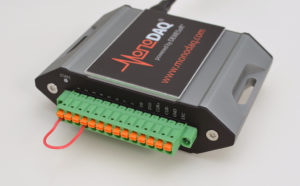When the channels are set up, we can enter Measure mode. On the top left part of the screen we have a control knob and input field for setting the analog output voltage. We added two recorders to show the measured voltage over time: the top one’s Y axis is scaled to show the full input range of the “Voltage In” channel which is +-10 V. The bottom one is autoscaled to the signal and its X axis is set to a shorter of a few seconds. We also added three digital meters on the top right showing the actual, average and ACRMS values of the measured voltage. We set the voltage output command from 0 V to 2 V and took a screenshot below. The above recorded shows the step in voltage while the bottom one is zoomed in and basically shows the noise level of the signal.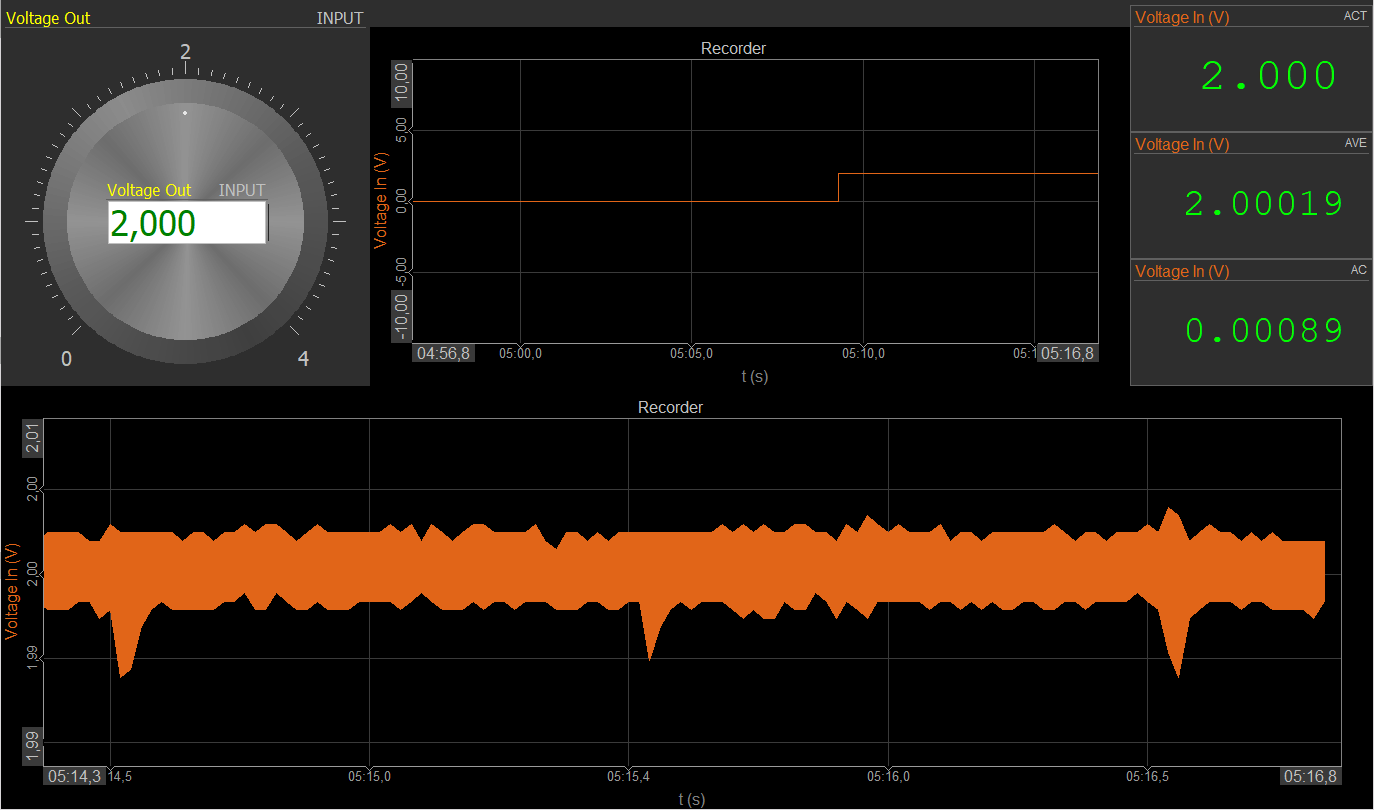Now obviously we are measuring 2.000 V back but let’s focus on the ACRMS value of the Voltage In channel (the bottom digital meter). It shows 0.89 mV. That is the RMS value of the noise in the signal, calculated in blocks of 0.5 seconds. Calculating the AC RMS value means taking away the constant (DC) component of the signal and only taking into account the changing (AC) part of the signal. While DEWESoft offers us those values by simply choosing the type of display in digital meter settings, we can also calculate those parameters in separate math channels.

To “remove the DC component” from the signal in practice means that we apply a high-pass filter to it. This is done in the Math section of DEWESoft Channel setup in the IIR Filter tab. If we want the oscillations of 1 Hz to be attenuated by 100 dB with a 6th order Butterworth filter, we need to set the cutoff frequency to 7 Hz. This means that if the signal is oscillating at 1 Hz and a peak-to-peak value of 1 V, the peak-to-peak value of this oscillation will be 10 uV (100 dB means the ratio of 10^5=100,000). Lower frequencies will be attenuated even more. Given the approximately +-1 mV of AC nosie that we are seeing in the recorder, this filter will sufficiently remove the lowest frequencies from the signal.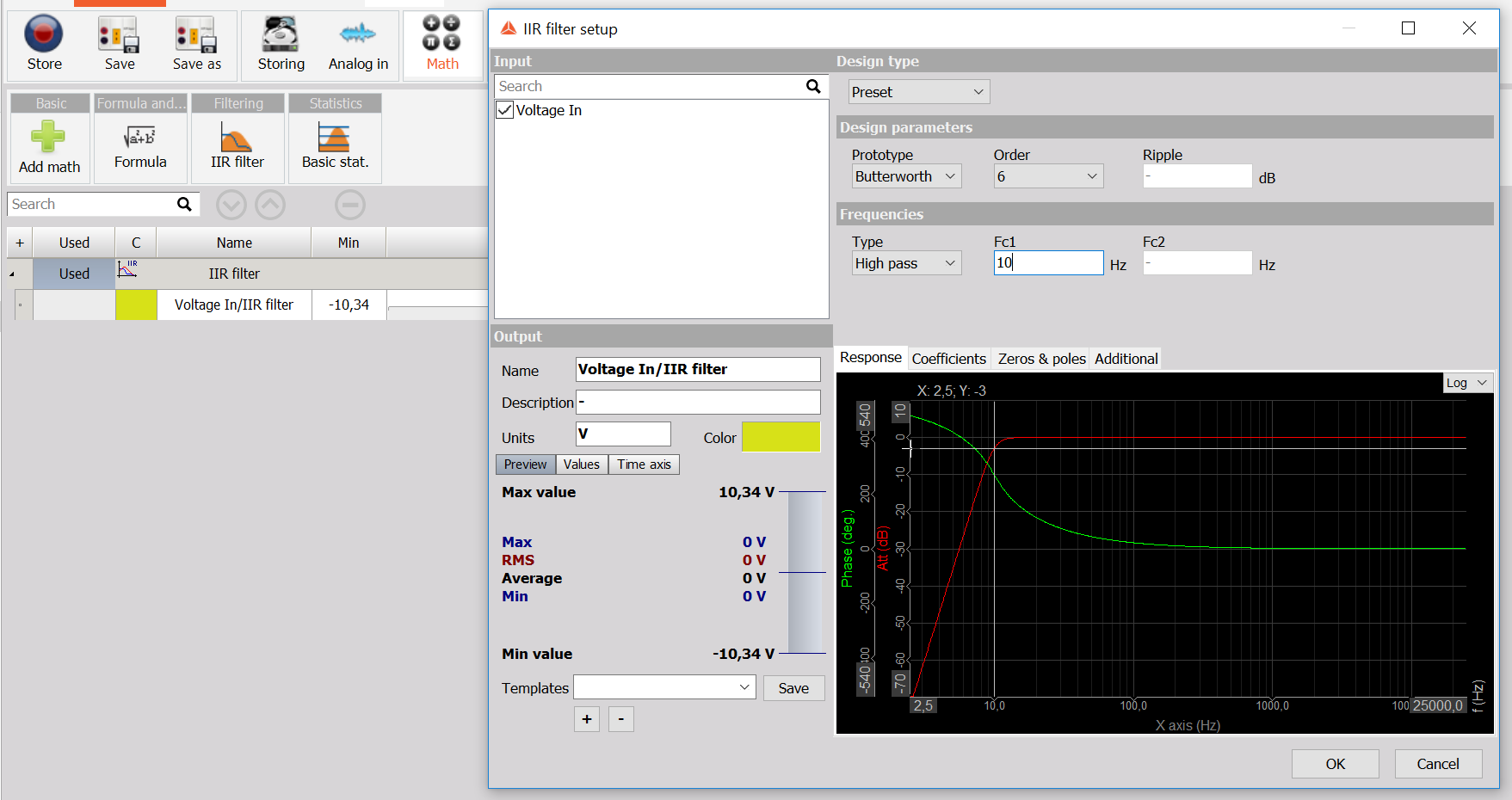The actual sample-by-sample value of the filtered signal does not tell us much about the noise. We need to calculate the RMS value over a block of time. This is done with the Basic statistics module in DEWESoft Math. It allows us to use the previously created Math channel (Voltage In/IIR Filter) in a further calculalation. This way we create a Voltage In/IIR Filter/RMS channel that should match the AC RMS value shown in the digital meter.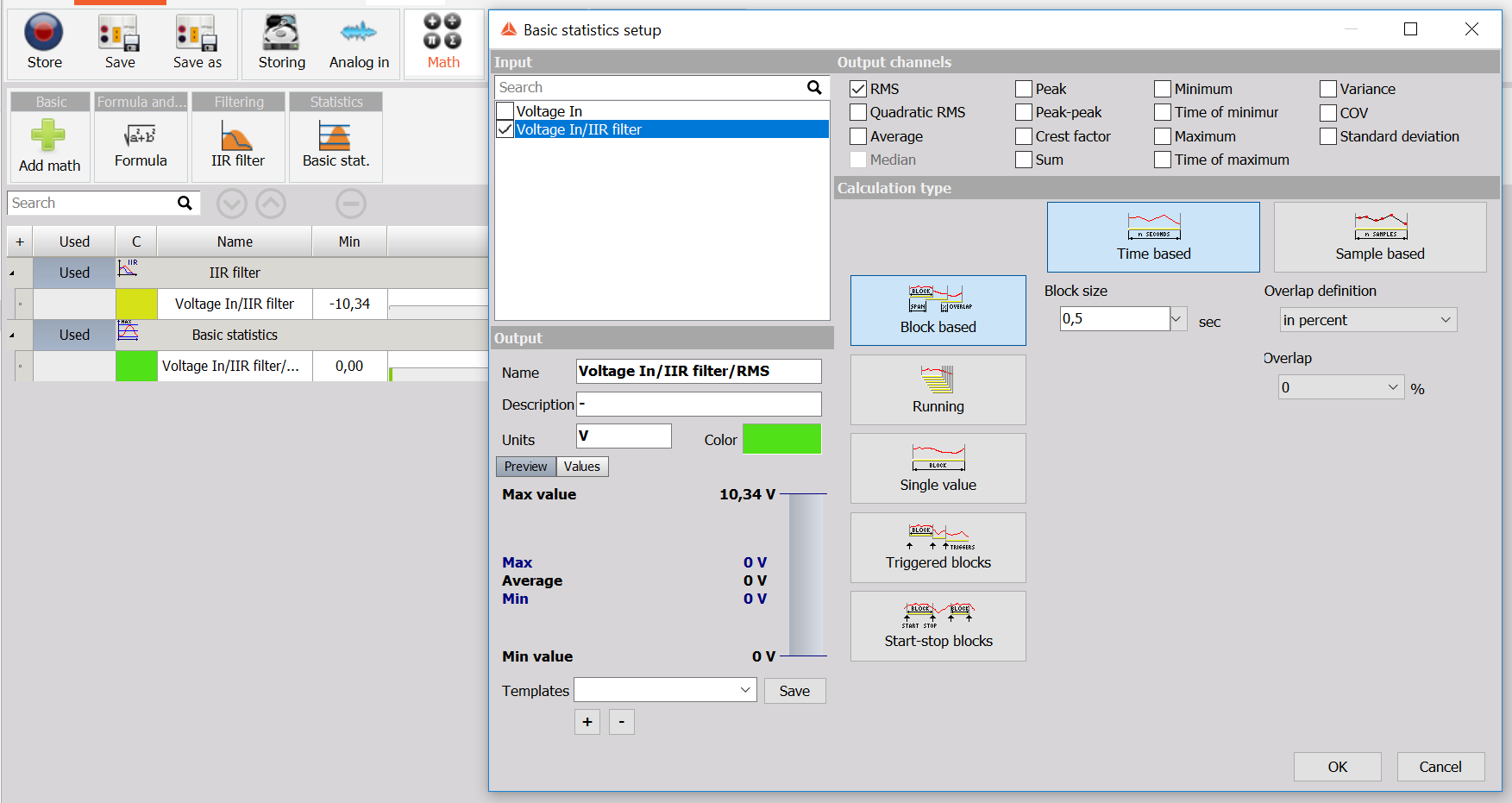Going to Measure mode we can check that the AC RMS value of the Voltage In channel approximately matches the calculated Voltage In/IIR Filter/RMS channel. We can use this channel to calculate the signal to noise ratio (SNR) of the signal. We now have the noise component, but still need to calculate the “signal” component. Since we are interested in measuring the DC component of the Analog Out channel, we can filter the Analog In channel with a low pass filter to remove all the high frequency noise. Let’s choose the same Butterworth filter as before but set it to Low Pass and a cutoff frequency of 5 Hz. Finally we can use both filtered channels in a formula calculating the SNR in dB.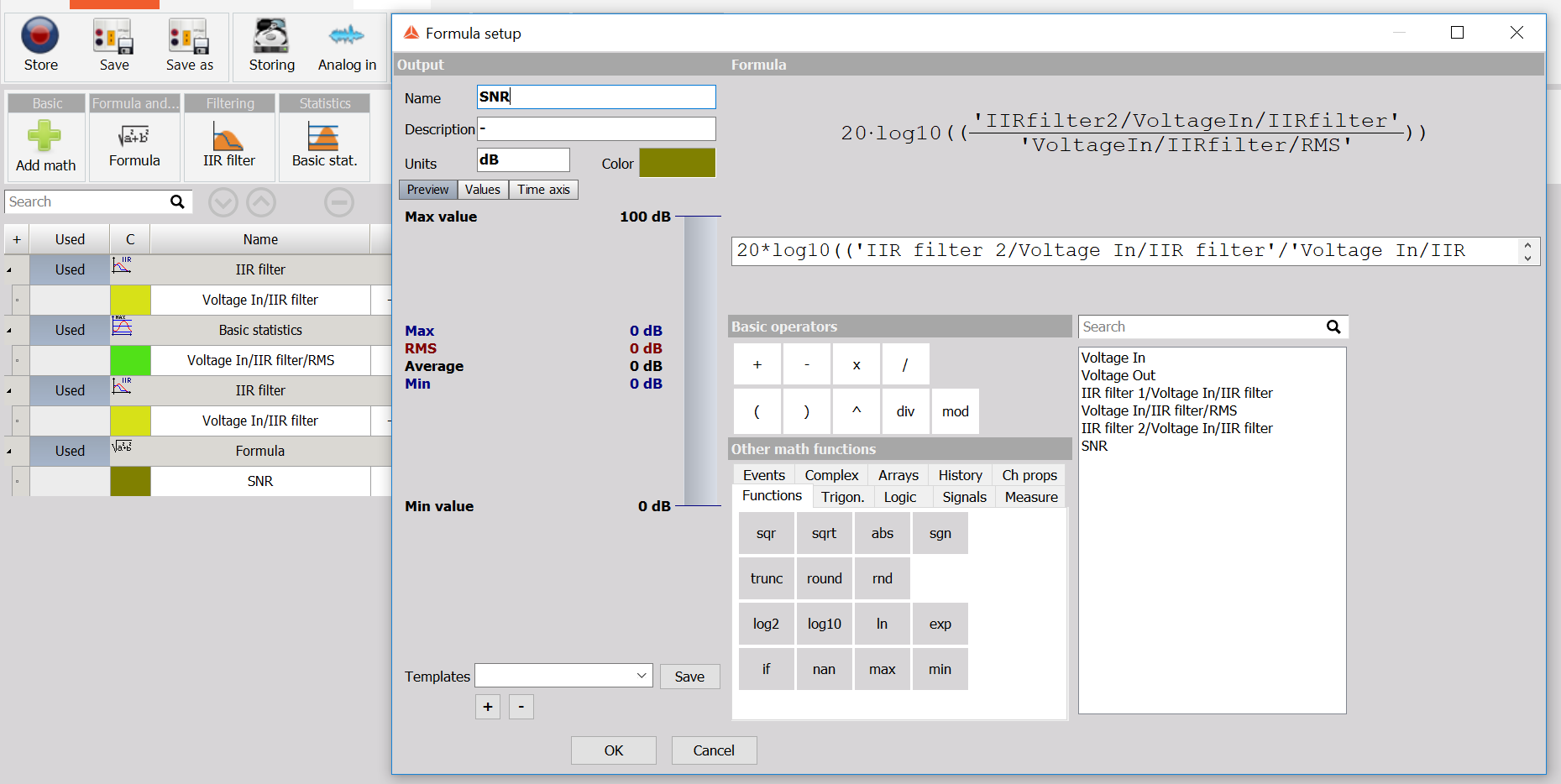Going into Measure mode we can add the SNR formula value next to our digital meters to have all the values in the same place.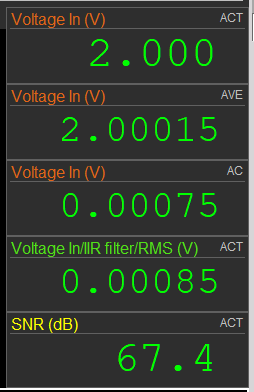Calculating the SNR when the signal of interest is a constant DC value is straightforward. But what if we are interested in the SNR of a 1 kHz sine wave? In that case we cannot just apply a low pass filter at 5 Hz, because that would remove our signal at 1 kHz. It is first useful to look at the signal in frequency domain, which is quickly displayed in DEWESoft with an FFT display. The signal has a relatively meaningful amplitude only at 1 kHz while all the rest is noise. A simple way to compute an approximation of the SNR of that signal is to apply a band pass and band stop filters, take RMS of both of them and use them in the SNR formula. Try it out yourself!